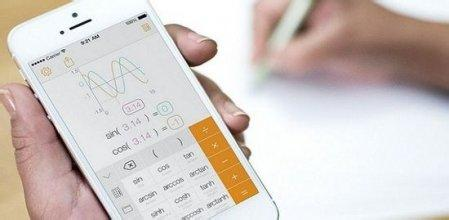# 新闻动态

1、库房耗冷量，即在单位时间里必须从库房内取的热量，根据产生的热量不同，库房耗冷量可分为五类。

Q1—围护结构传热量W；

Q2—货物热量 W；

Q3—通风换气热量W；

Q4—电动机的运转热量 W；

Q5—操作热量 W2、库房冷却设备冷负荷计算公式为：

Qq = Q1+ρQ2 + Q3 + Q4 + Q5

Q1—围护结构传热量W；

Q2—货物热量 W；

Q3—通风换气热量W；

ρ—负荷系数；冷却间和冻结间的负荷系数ρ取1.3，其他库房取1；

Q4—电动机的运转热量 W；

Q5—操作热量 W

3、冷库外墙、屋顶、地面保温层结构

4、计算各面传热层系数

—围护结构内侧表面传热系数。

—各层隔热材料的导热率。

4.1冻结间

（1）无防风设施的外墙传热系数：

k=1/(1/23+0.37/0.8141+0.15/0.0314+1/29)=0.19W/(m²℃)

k=1/(0.37/0.8141+0.15/0.0314+1/29)=0.19W/(m²℃)

（2）地面传热系数：

k=1/(1/8+0.125/1.5468+0.0002/0.0465+0.25/0.043+0.0002/0.047+0.02/0.9304+1/29)=0.16 W/(m²℃)

（3）顶层传热系数：

k=1/(1/23+0.02/0.9304+0.25/0.0314+0.02/0.9304+1/29)=0.12 W/(m²℃)

4.2冻结物冷藏间

（1）无防风设施的外墙传热系数：

k=1/(1/23+0.37/0.8141+0.15/0.0314+1/8)=0.19W/(m²℃)

（2）地面传热系数：

k=1/(1/8+0.125/1.5468+0.0002/0.0465+0.25/0.043+0.0002/0.047+0.02/0.9304+1/8)=0.16 W/(m²℃)

（3）顶层传热系数：

k=1/(1/23+0.02/0.9304+0.25/0.0314+0.02/0.9304+1/8)=0.12 W/(m²℃)

5、设备负荷计算

（1）围护结构传热量Q1：

—围护结构温度修正系数。

﹑—围护结构内﹑外侧温度。

（海南市）， = -23℃ （冷库内）

= 0.19×A1×1.05×(31-(-23)) = WQ1X(W)

= 0.12×FWMm²×1.2×(31+23)=FWMX(W)

=  0.16×FDMm²×0.7×(31+23)=FDMX(W)

（海南市）， = -18℃ （冷库内）

= 0.19×A1×1.05×(31-(-18)) = WQ1X(W)

= 0.12×FWMm²×1.2×(31+18)=FWMX(W)

=  0.16×FDMm²×0.7×(31+18)=FDMX(W)

Q=WQ1X(W)+WQ2X(W)+LCTX(W)+FWMX(W)+FDMX(W)+WQ1X(W)+WQ2X(W)+LCTX(W)+FWMX(W)+FDMX(W)=QTZFHX(W)

（2）货物热流量Q2计算

Q2=Q2a+Q2b+Q2c+Q2d

Q2=

Q2b—包装材料和运载工具热流量。

Q2c —食品冷加工过程的呼吸热

Q2d—食品冷藏过程的呼吸热

—冷间的每日进货量。

— 货物进入冷间初始温度时的比焓。

—货物在冷间终止降温时的比焓。

—货物冷加工时间。

—货物包装材料或运载工具质量系数。

—包装材料或运载工具的比焓。

—包装材料或运载工具进入冷间时的温度。

—包装材料或运载工具在冷间内终止降温时的温度。

q1 q2—鲜果、蔬菜冷却初始、终止温度时的呼吸热w/kg

Gn—冷却物冷藏间的冷藏量,代入数据：

=[RJHLXXXX×(318-12.2) /20 +RJHCSXX×0.1×CJHLXXX×0.42×（35+23）/20]/3.6=Q2w

=[RJHLXXXX×(318-12.2) /20 +RJHCSXX×0.1×CJHLXXX×0.42×（35+23）/20]/3.6=Q2w

（3）由于所计算的是冻结物冷藏间和冻结间没有呼吸热和通风唤气所以不需要。

（4）冻结间电动机运转热量Q4

—电动机额定功率W

—热转化系数，在冷间内取1

—电动机运转时间系数

（5）冻结物冷藏间操作热流量计算Q5

Q5=

—每日开门换气次数取次，取次。

—空气幕效率修正系数，可取，如不设则取。

—库房空气密度，。

—操作人数,可按库房内公称容积,每增加人，取4人。—每个操作人员产生的热量。

 温度回路 房间 -33 冻结间 1 1 – – 1 – – 1.07 -28 冻结物冷藏间 1 0.5 – – 0.5 – 0.5 1.07QQ在线咨询
400服务热线
400-892-0198

13166094839